The core math topics covered in grade 1 typically are:

Numbers and Operations

• Counting up to 120
• Place value: tens and ones
• Use place value to add and subtract
• Word problems within 20
• How adding and subtracting relate to each other
• Add and subtract within 20
• The meaning of the equal sign
• Working with equations

Geometry

• Describe shapes
• Circles and rectangles into parts

Measurement

• Measure length
• Tell time
• Organize objects

You can find greater detail in the Common Core Math Standards for Grade 1.

## Free grade 1 math worksheets

Our grade 1 math worksheets extend significantly beyond core topics and are divided into 13 subject areas:

 Number Charts & Counting Fractions Number Patterns Measurement Comparing Numbers Counting Money Base 10 Blocks Telling Time Place Value Geometry Addition Word Problems Subtraction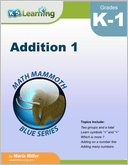Addition 1 The concept of addition and addition facts within 0-10. Introduces number lines and symbols for addition.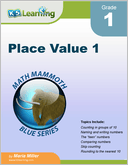Place Value 1 Covers 2-digit numbers, with emphasis on "tens and ones". Includes skip counting, rounding to the nearest 10 and adding / subtracting tens.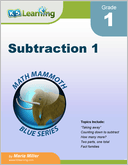Subtraction 1 Concept of subtraction and subtraction facts within 0-10.  Includes "taking away", counting down and fact families.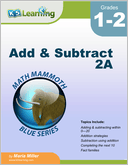Add and Subtract 2A Adding and subtracting within 0-20, adding doubles, subtraction strategies, completing the next ten, fact families and single digit subtraction facts.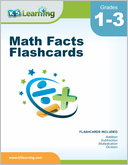Math Facts Flashcards Flashcards for addition, subtraction, multiplication and division facts, 0-12.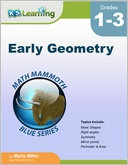Early Geometry Basic shapes (rectangles, squares,...), right angles, symmetry, mirror points, perimeter and area.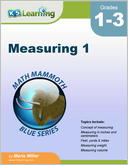Measuring 1 Length, weight and capacity for grades 1-3, including the concept of measuring lengths, weights and volumes.  Both standard and metric systems are used.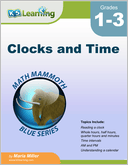Reading a clock, time intervals, am & pm and understanding a calendar.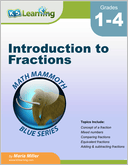Introduction to Fractions Introducing fractions, mostly for grades 3-4. Concept of a fraction, mixed numbers, comparing fractions, equivalent fractions, adding and subtracting fractions and fractions multiplied by a whole number.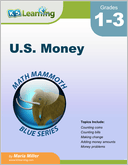U.S. Money Recognizing coins and bills; counting money, making change and money problems. We also have similar books in other currencies (Canadian money, Australian Money, British Money, European Money and South African Money).